# ISEE Lower Level Math : How to find the area of a triangle

## Example Questions

1 2 3 5 Next →

### Example Question #317 : Plane Geometry

Find the area of a triangle with a base of 7in and a height that is two times the base.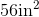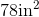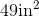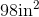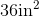Explanation:

To find the area of a triangle, we will use the following formula: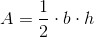where b  is the base and h is the height of the triangle.

Now, we know the base of the triangle is 7in.  We also know the height is two times the base.  Therefore, the height is 14in.

Knowing this, we can substitute into the formula.  We get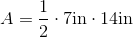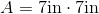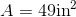### Example Question #318 : Plane Geometry

Find the area of a triangle with the following measurements:

• height:  5in
• base:  6in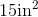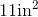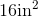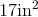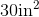Explanation:

To find the area of a triangle, we will use the following formula:where b  is the base and h is the height of the triangle.

Now, we know the base of the triangle is 6in.  We also know the height of the triangle is 5in.  So, we get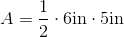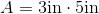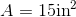### Example Question #43 : How To Find The Area Of A Triangle

Use the following triangle to answer the question: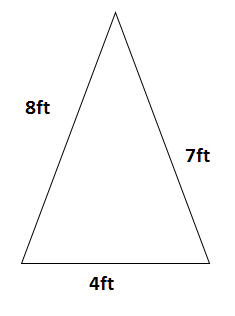If the triangle has a height of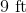, find the area.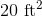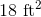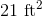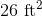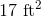Explanation:

To find the area of a triangle, we will use the following formula: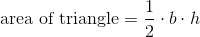where b is the base and h is the height of the triangle.

Now, given the trianglewe can see the base is 4ft.  We also know it has a height of 9ft.

Knowing this, we can substitute into the formula.  We get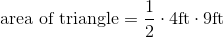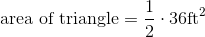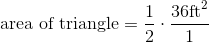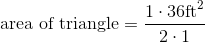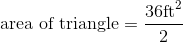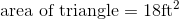1 2 3 5 Next →

### All ISEE Lower Level Math Resources